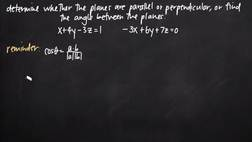PLAY PREVIEW

### Segments in this Video

#### Perpendicular Planes(02:39)

FREE PREVIEW

Perpendicular Planes are discussed in this section.

#### Parallel Normal Vectors(01:12)

If the equation is true, then the planes are parallel. If the equation is not true, then the planes are not parallel.

#### Concluding Planes are Perpendicular(01:28)

To test whether or not two vectors are perpendicular, take the dot product of the vectors. If the dot product is equal to 0, then the vectors are perpendicular to one another.

#### Revealing Angle Between Planes(02:17)

Perpendicularity is a condition in which vectors are oriented at right angles to one another, and perpendicularity can be determined using the dot product.

#### Theta Problem: Angle Between Planes(01:39)

How to find the angle between two planes is discussed in this section.

#### Credits(00:00)

Credits

For additional digital leasing and purchase options contact a media consultant at 800-257-5126
(press option 3) or sales@films.com.

# Parallel, perpendicular and angle between planes

Part of the Series : Integral Calc: Calculus 3
 3-Year Streaming Price: \$49.95

Share

### Description

This video tutorial works through math problems/equations that address topics in Calculus 3, Partial Derivatives. This specific tutorial addresses Parallel, perpendicular and angle between planes.

Length: 10 minutes

Item#: FMK275736Closed Captioned